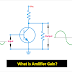The concept of gain of an amplifier is very important, you must have a clear knowledge about it because the term 'Amplifier Gain' will frequently come when you going to read something about an Amplifier.

An amplifier is an electronic circuit that can amplify a signal by taking an external voltage. An amplifier can produce output signals same as the input signal but in larger magnitude.

So each amplifier has a limitation that how much it can amplify a signal. An amplifier can't amplify a signal up to infinite value.

## What is the Amplifier Gain?

So, in simple words, the ability of an amplifier to amplify a signal is called The gain of that amplifier.

The gain of an amplifier is the ratio of output to input.

The output signal of an amplifier may be voltage controlled or current controlled, so the gain also measured with current or voltage. So you may heard about both terms - Current Gain and Voltage Gain.

For example, the ratio of output current to the input current of an amplifier is called the Current Gain of that amplifier.

And, the ratio of the output voltage to the input voltage of an amplifier is called Voltage Gain of that amplifier.

Similarly, the ratio of output power to the input power of an amplifier is called the power gain of that amplifier.
For certain amplifiers, the ratio of changes in the output signal with respect to the changes in the input signal is called the gain of that amplifier. In this case, the gain can be calculated by taking the ratio of RMS value of output and input signals.

## Importance of Amplifier Gain

1. The gain of an amplifier indicates that how much it can amplify a signal.

2. It indicates the ability of an amplifier to amplify a signal.

3. It indicates the effects of feedback from output to input.

4. It indicates the efficiency and range of an amplifier.

## Measurement and Calculation of Amplifier Gain

As the gain of an amplifier is just a ratio, so it does not have any unit. But in some cases, it is measured with a logarithmic scale and that time it has a unit which is called the decibel. 1 bel is equal to 10 decibels.
Suppose we want to calculate the voltage gain of an amplifier which having input voltage 5V and output voltage 15V.

So, the voltage gain will be (Vout/Vin) = (15/5) = 3

When two amplifiers are connected in a cascade manner then the overall gain of the system will be the multiplication of their individual gains.

For example, two amplifiers are connected in a cascade manner and they have gain 10 and 15 respectively.

So, the overall gain of the system will be, 10 x 5 =50

## Why Gain of an amplifier reduces with increasing in Frequency?

Most of the amplifier's load is the parallel combination of the resistor and capacitor.
So when frequency increases the impedance of the capacitor used in the amplifier circuit will decrease, so more current will flow through the capacitor instead of the resistor. Hence the voltage across the load resistor will decrease. So the output voltage will low for the same input voltage when the frequency is increased. As the output voltage getting low the gain of the amplifier also be reduced. High frequency and high power amplifiers very larger in size.

Thank you for visiting the website. keep visiting for more updates.

Gain of Amplifier, Types, Importance, Effects, MeasurementReviewed by Author on January 28, 2020 Rating: 5